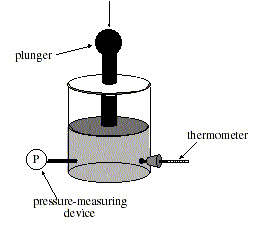JEE 2006 Sample Question Paper : Page 1 | Page 2 | Page 3 | Page 4 | Page 5 | Page 6 | Page 7

# IIT JEE 2006 Sample Question Paper

Passage 6

The relationship between pressure and volume of a gas was studied while the temperature was held constant. A container was filled with helium gas at room temperature and sealed (see figure below). The pressure on the gas could be controlled by varying the force exerted on the plunger, and the volume could be determined by the height of the plunger.
(Note: Assume that the mass of the plunger is insignificant.)Experiment 1
The initial pressure in this experiment was 1 atmosphere (atm). At that pressure the gas occupied 1 liter (L). The pressure of the helium was increased very slowly, by decreasing the volume, so that the gas remained at room temperature. The heat generated was released into the surrounding atmosphere. The pressure and the volume of the helium were recorded in Table

Table 1.
Pressure
(atm)
Volume
L
1.01.00
1.50.67
2.00.50
2.50.40
3.00.33

Experiment 2
Experiment 1 was repeated at room temperature with an initial pressure of 1 atm and an initial volume of 2 L. The results are shown in Table 2.

Pressure
(atm)
Volume
L
1.02.00
1.51.33
2.01.00
2.50.80
3.00.67

Experiment 3
In this experiment the entire gas container was insulated to prevent heat loss. The procedures from Experiment 1 were repeated. It was observed that the temperature of the gas rose as the volume decreased. Table 3 shows the volume measured at each pressure during the compression.

Pressure
(atm)
Volume
L
1.01.00
1.50.78
2.00.66
2.50.58
3.00.52

The insulation was then removed and the pressure maintained at 3 atm. As the gas cooled to room temperature, the volume of the gas slowly decreased from 0.52 to 0.33 L.

Q. 27 How is the design of Experiment 1 different from that of Experiment 2?

1. The container is insulated in Experiment 2, but not in Experiment 1.
2. A different gas is used in Experiment 2 than in Experiment 1.
3. The initial volume of the gas in Experiment 1 is half that of Experiment 2.
4. The initial volume of the gas in Experiment 1 is twice that of Experiment 2.

Q. 28 A seventeenth-century scientist named Robert Boyle discovered that as the pressure on any confined gas increases (with the temperature held constant), the volume decreases. The best way to verify these results would be to repeat Experiment 1 with:

1. an identical container made of a different material than the original.
2. an identical volume of water.
3. several different gases.
4. an unsealed container.

Q. 29 If Experiment 1 is continued and the pressure is increased to 4 atm and remains fixed at this pressure, the gas would occupy a volume of approximately:

1. 0.25 L.
2. 0.33 L.
3. 0.50 L.
4. 1.00 L.

Q. 30 If Experiment 1 had started with 0.5 L of gas at 1 atm, what volume would be recorded when the pressure was 2.5 atm?

1. 0.20 L
2. 0.30 L
3. 0.40 L
4. 0.44 L

Q. 31 Which of the following statements best explains why the temperature of the container decreased after the insulation was removed in Experiment 3?

1. The pressure increased, causing the temperature to decrease.
2. The volume decreased, causing the temperature to decrease.
3. Heat flowed from the surrounding atmosphere, through the container, and into the gas.
4. Heat flowed from the gas, through the container, and into the surrounding atmosphere.

Q. 32 Suppose that Experiment 3 was modified so that the insulation was removed after each pressure increase and the container was allowed to sit at that pressure until its temperature cooled to room temperature. How would the volume readings be influenced, if at all, by this modified procedure?

1. They would be the same as those in Experiment 1.
2. They would be the same as those in Experiment 3.
3. They would be greater than those in Experiment 2.
4. They would be smaller than those in Experiment 1.IIT JEE - HomeWebsites of IITJEE SyllabusIIT Question PapersBooks For IITIIT JEE 2008IIT JEE 2007IIT JEE 2006IIT JEE 2005IIT CoursesSeats ReservationEligibility CriteriaAnalysis of IIT JEEIIT AlumniIIT JEE TipsIIT Articles